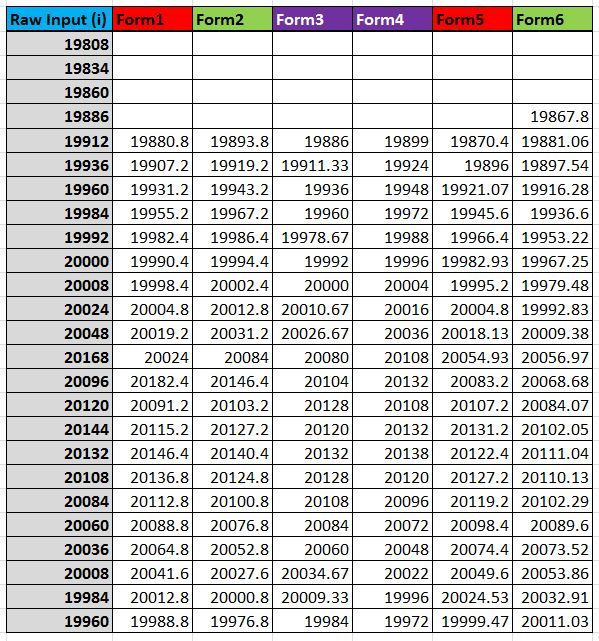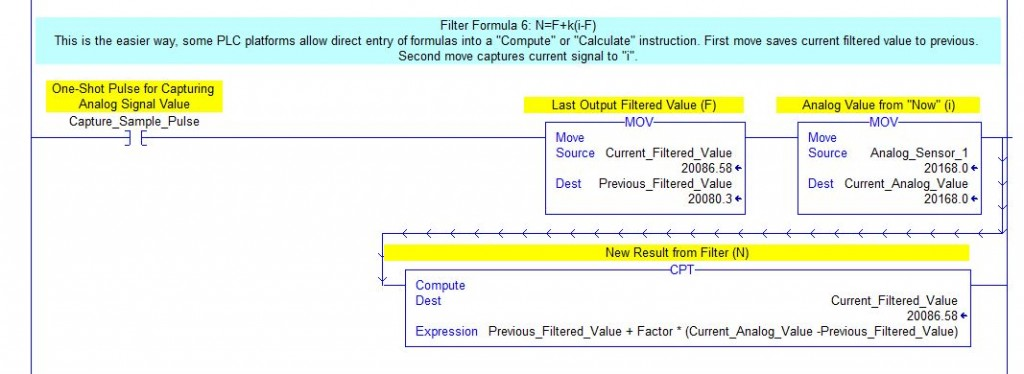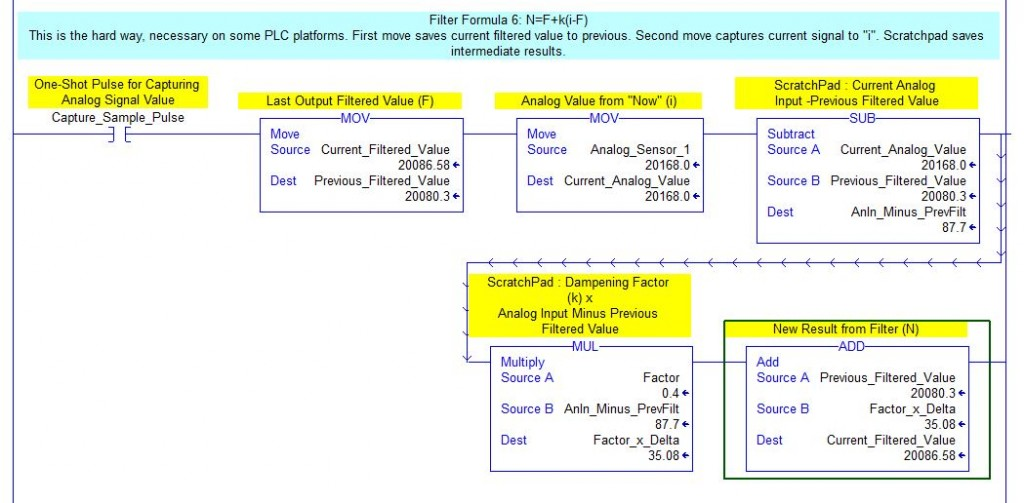# Ladder Logic 402: Data Manipulation and Filtering

In last weeks’ post, I discussed acquiring data using a PLC to capture values from an analog card. I showed some fairly straightforward methods of capturing data using FIFO methods, averaging data values, and how some of the ladder logic might be performed on different PLC platforms.

This post discusses some of the methods of filtering data and ways to simulate a filter using Microsoft Excel before you even code it in the PLC.

First I did some searching online to see what kind of filtering algorithms people are already using on a PLC. I found a variety of posts on different forums including plcs.net, plchowto.com, and control.com. There were also responses and recommendations on Linked In forums, and even a new one posted here in last weeks comments.

First the formulas: I have used the same variable names for all formulas even though they differed extensively in the various posts. Here I use the following:

N = New value calculated and substituted for the input value. In other words, the calculated filtered value.
L = The previous captured input value.
F = The previous calculated filtered value.
k = The manipulated factor for all calculations (sometimes known as Alpha).
Avg = A running average over x number of previous samples, I used 3 for x in this example.

The formula for a first order filter is New Filtered = Last Filtered + Factor * (Input – Last Filtered).
This equates to N=F+k(i-F) according to the variables I listed above. The actual formula uses Alpha instead of k. Results from this formula are listed as Formula 6 below in my simulation.

To simulate a signal I listed some analog values in one column on an Excel spreadsheet. These values are typical for a 13 bit signed analog signal from a PLC card; notice that they increment or decrement by a factor of eight. This would mean that on a 0-10v or 0-20mA signal with a range of 0-32,767, there would be 4096 possible values for the signal. The other half of the range, -32768 to -1, is not obtainable from the field device.The first column shown above are the input values I entered. The value of 20168 simulates a big noise spike that you might want to filter for, similar to that described by Quy Phan in his question. The following columns show results after using the various filtering formulas I found during my search. The reason the input column has earlier values in it is so I could use a running average of up to five values in my formulas. Formula 6 (the one I typically use) also needs to use the previous filtered value, so that’s why it has an extra value in it also.This is a key with the variables I listed and the formulas. The k values are linked to cells used in the table, so I can change them as needed.

These are the first three formula’s results

and these are the next three. As you can see, Formula 1 actually looks like it makes the signal worse. This one was listed on plcs.net; I tried messing with brackets and the k value but it didn’t seem to help. In the description the writer said that k was the number of samples to filter across, but any positive integer was a disaster, so I gave up.

Formula 2 and Formula 4 did nearly the same things; in the instructions Formula 2 was “k=1.0, no filtering, k=0, output freezes”. Formula 4’s instructions said “k=1.0, no filtering, 2.0, 4.0 and 8.0 give increasing amounts of filtering with more dampening, but more delay”.

Formula 3 is a straight running average, and seems to actually work pretty well. I used the current and previous two values for an average of three, when using more there was more lag.

Formula 5 also uses a running average. I used three samples in the average also, but I believe the intent was to use four or five. When I did, the delayed reaction (lag) got worse.

As I mentioned, the formal definition of a first order filter is what I have used in the past. This is represented by Formula 6. Sometimes this is known as a first derivative filter; if the points are equated to position, this would represent a velocity based filter. A second order filter would then represent Accel/Decel and a third order filter would represent “jerk”. The filters would work off of the delta between the current and previous values. I don’t know a lot about process control, so I’m not sure how the second and third derivatives might relate to flow or temperature.

On last week’s post, Karl Newman described a simple moving average filter with a formula suggestion. I wanted to get this post completed before heading off to Los Angeles this week, so I didn’t get a chance to try it. He says that it has no data storage requirements, but of course to calculate new or obtain old averages, you have to retain previous averaged values. I intend to try it out on my spreadsheet, but hopefully Karl will let me know what he meant by no data storage.

The main point I hope to get across with this post is that you can simulate formulas very easily in Excel, you may want to check things out before coding it into the PLC as illustrated by Formula 1.

Putting a formula into PLC form will vary a lot between platforms. In ladder logic, a “calculate” type command lets you type the formula in directly, though you may still need to use FIFOs as described last week, especially when averaging. Structured Text is also a good option if your platform has it. Siemens STL (Statement List) also makes it easier.

Again, Quy Phan’s task is going to be a bit tougher and take more time with his Automation Direct DL205. There is a lot of LOAD and OUT necessary when using stack based logic and math. He will also need to determine a proper time constant for data acquisition.

I may address what it takes to turn these formulas into ladder at a later date. As this is one of my first advanced topics, most of you with experience already know how to do that, but for those just learning it could be a useful exercise.

*** Hello from Sunny Los Angeles, 14 March 2016! I am updating this post with a bit of ladder as I mentioned last weekend. This uses Formula 6 from the spreadsheet.

Below is the easy way, if your software has an instruction that allows you to enter a full formula:And here is the slightly more difficult way, doing each mathematical instruction sequentially and saving values in “scratchpad” intermediate values. Still not too bad…Once again, this is in response to Quy’s original problem of filtering on an Automation Direct DL205. Quite a few things to add to this code on that platform.

1. Addresses are not tags, they are 16 bit octally numbered registers, like V7020-V7027. Since most math in my filter example uses REAL or Floating Point numbers, the analog value will have to be converted from Integer form to REAL, using various intermediate registers. REALs of course fill two 16 bit addresses each, so be careful about reserving space.

2. Math and move functions won’t look like this, they will require LOAD Double Vxxx and OUT Double VXXX commands. Instructions will not be able to be placed in series, but instead must be either in parallel or use separate rungs.

3. Timers run on a 100ms time base, so it will be difficult to sample repetitively and accurately. See last week’s post for why this may be a problem.

Also gathering data to analyze for your spreadsheet will be more difficult coding. Ideally you would sample at 10-20ms to analyze your signal, but filter at a slower rate, say 100-200ms.

Its funny, I put this on some Linked In forums a couple of days ago and have gotten some interesting responses, like “MS Excel is not compatible with Ladder Logic”. Hmmm… not sure the post was read correctly, I guess that’s why I put it in the 400 series. Sorry about that. Excel is just used as an analysis tool here.

Though just for fun, check out this post from several years ago, actually you can write importable code in Excel! In AB, XML files are directly importable and easily converted from an L5X file to ladder, while in Siemens, it can be imported as STL (Statement List) and converted to ladder! I bet you can do that on some other platforms too.

I also got some more formula suggestions to try out. Keep them coming, as I mentioned, I am working on an advanced PLC programming book and appreciate the input!Electrical Engineer and business owner from the Nashville, Tennessee area. I also play music, Chess and Go.

1.Miguel says:

•Frank says:

Yes. The ControlLogix platform has individual hardware filters for each analog point; you can filter 50-60Hz and also based on the sampling rate.

•Mohamed Lakhdari says:

Greetings,

Thank you for a great job on explaining filters in a good and understandable way.
By the way per Siemens Tech Support STL is an IL (Instruction List Language) while SCL (Structured Control Language) is the Siemens Version of ST

2.kurt klingbeil says:

I almost always use OUT = (IN + LAST(N-1))/N
translated into Excel-ese: =(D12*(\$H\$4-1)+C13)/\$H\$4

or in anachronistic Ladder-ese, with the capability of dynamic filter constant adjustment:
SUBTRACT (N ,1 )-> M
MULT(OUT, M) -> OUTM